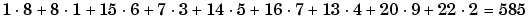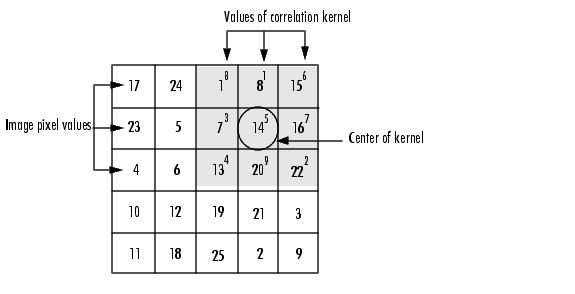Image Processing Toolbox User's GuideCorrelation

The operation called correlation is closely related to convolution. In correlation, the value of an output pixel is also computed as a weighted sum of neighboring pixels. The difference is that the matrix of weights, in this case called the correlation kernel, is not rotated during the computation. The filter design functions in the Image Processing Toolbox return correlation kernels.

The following figure shows how to compute the (2,4) output pixel of the correlation of `A`, assuming `h` is a correlation kernel instead of a convolution kernel, using these steps:

1. Slide the center element of the correlation kernel so that lies on top of the (2,4) element of A.
2. Multiply each weight in the correlation kernel by the pixel of `A` underneath.
3. Sum the individual products from step 3.

The (2,4) output pixel from the correlation isComputing the (2,4) Output of CorrelationConvolution Filtering Using imfilter